|

# 这就是中国第一个装配化内装商品住宅

随着国内《商品住宅装修一次到位实施细则》的广泛推进实施，全国的商品住宅市场全装修交付已然成为大势所趋，国内各大企业在住宅全装修领域的探索也获得了许多积极的进展。

始终坚持“为爱定义生活”的景瑞地产与品宅精诚合作，秉承可持续发展理念，把位于青浦的城中公园项目，作为装配化内装科技领先实践的典范，打造出中国第一个装配化内装商品住宅项目，吹响了全装修时代内装工业化的嘹亮号角。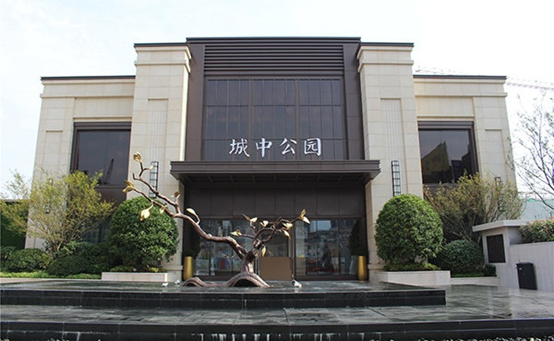▧ 项目名称：景瑞城中公园

▧ 项目类项：高端住宅

▧ 项目地点：上海·青浦

▧ 室内设计：品宅·品装设计机构(PPD)

▧ 工程施工：品宅·集栋工程

▧ 总建筑面积：33095㎡

▧ 总户数：328

▧施工周期：90天

家是人的居所，是围绕人的生活基本需求逐步建立起来的，为人的生活服务是设计的根本。

品宅跟随建筑设计标准，将关注点聚焦于建筑内部健康环境营造，秉持绿色、生态、健康的理念，在空气、水、光、声音、材料、温度、舒适性等多个维度上严格把控，指导设计的全过程。青浦区景瑞城中公园装配化内装商品住宅就具有突出表现，不仅在建筑品质上坚持高标准，更与品宅合作，实现内装工业化关键技术的应用，领航装配化内装商品住宅绿色健康的时代大方向。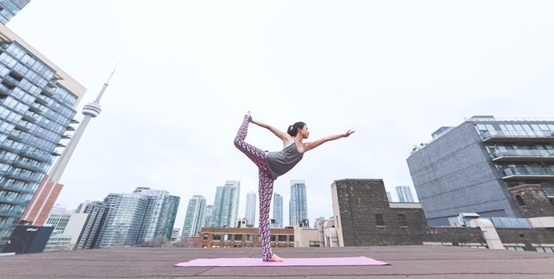中国的文化源远流长，伴随着太阳文化的诞生而生，接受光的照耀，热的温暖，山河的历练，自然的洗礼，人们的精神世界也和日渐富足的物质世界一样，越发丰盛而讲究。许多旧事物跟不上人们的需求而被替代，但是关于住所，人们始终在花费更多的心思去改变、去建造、去装扮，因为它不但是一个住所，更承载着家的温暖，心的归处，给致爱提供保护，让焦虑得意安放。品宅旗下品装设计机构(PPD)，把装配式科技内装技术应用于本案，改变长期存在的家装痛点不能满足人们对居住空间更高品质要求的矛盾。

生活的诗意在每一口新鲜的呼吸里，在每一次安静的门扇开阖中，在踱步于静谧书房的踏实里，在安睡于舒适闺房的甜梦中，是与家人共进晚餐的厨房，也是和朋友彻夜长谈的窗台。动与静，传统与当代，审美主义与实用主义，都可以在这个户型中得到充分兼容，达到平衡。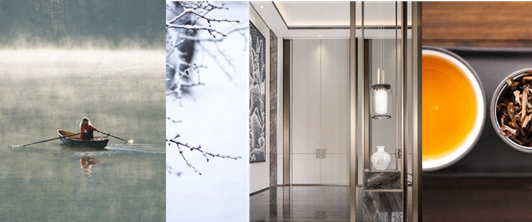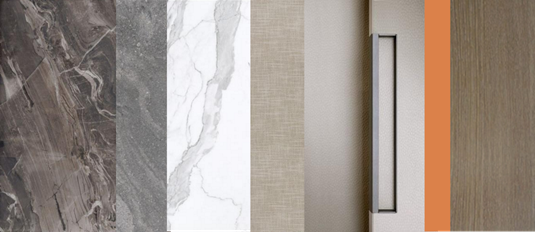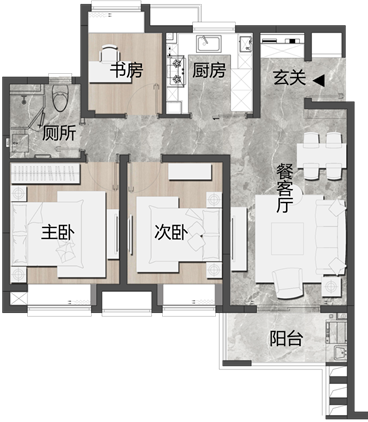△平面布局图

1、装配式墙面体系容纳你的休闲时光

青浦景瑞城中公园商品住宅采用的品宅的装配式墙面体系，通过调平构造，兼容各种原始建筑基层。面层采用无醛企口墙板，替代传统乳胶漆壁纸，无醛环保，确保居住者的健康，耐用耐擦洗，便于居住者日常维护，可以轻松局部维修，减轻物业维护负担。

装配化墙面体系可以叠加多样化的功能，在餐客厅区域，背景墙可搭配金属配件成为电视背景墙，镜面与装配化墙面的搭配，让客厅空间更有透气感和灵动性。

装配化的墙面体系可以实现空腔走线，因此无需预埋预留管线、点位，不破坏建筑墙面。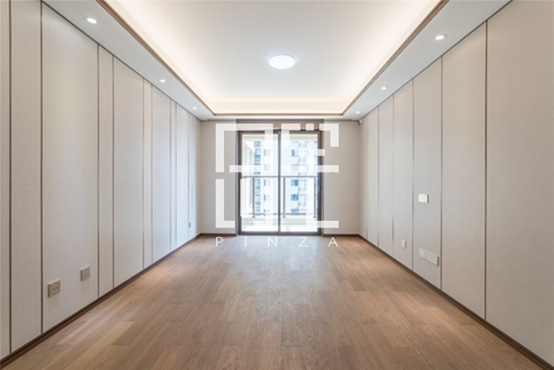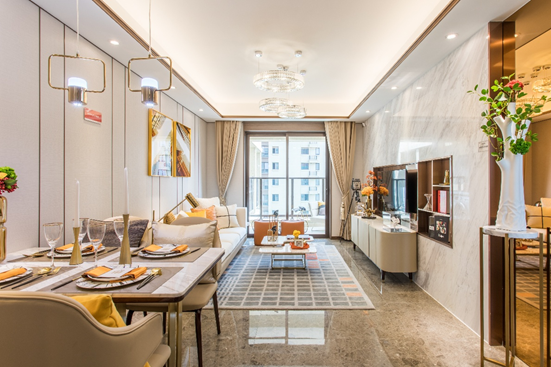△餐客厅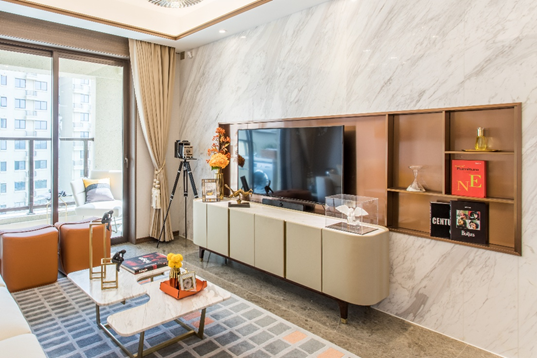△装配化客厅电视背景墙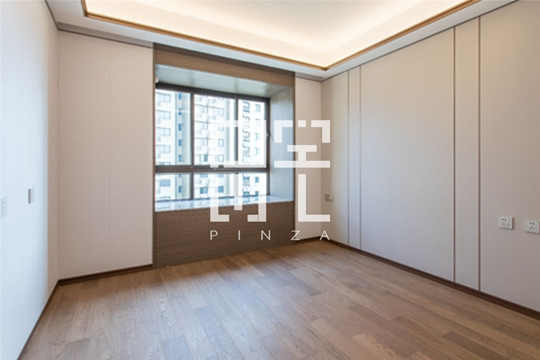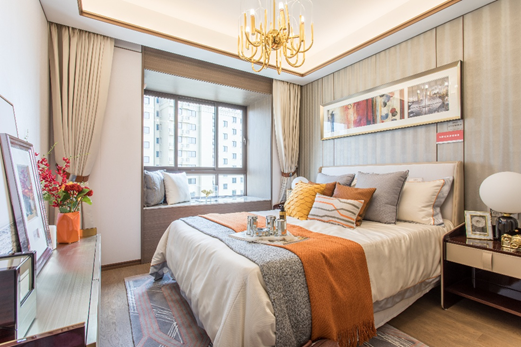△主卧

2、分体装配式厨房美食美刻的享受

品宅的分体装配式厨房为厨房空间提供了强大完备的解决方案，本案的开放式厨房墙面，采用不锈钢背板，持久抗污，经久耐用，便于居住者日常清洁打理。装配化的工艺完全杜绝了传统铺贴瓷砖空鼓脱落的问题。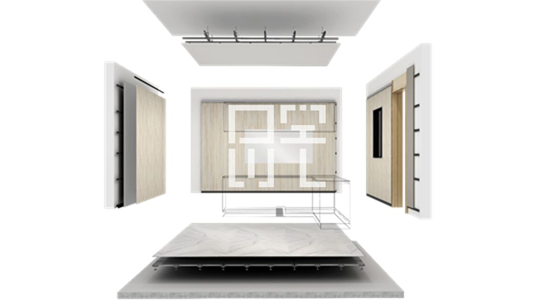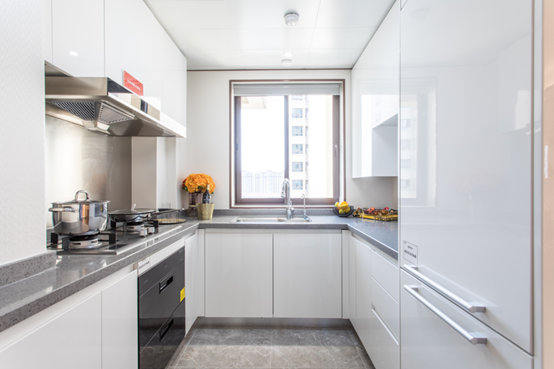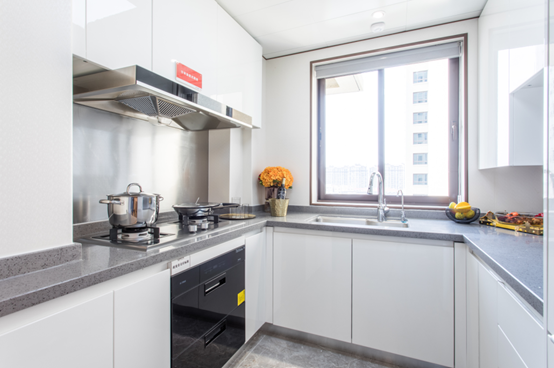3、分体装配式卫生间一年四季的清爽

品宅的分体装配式卫生间区别于整体装配式卫生间，最大的优点是可以适配各种建筑空间，高度匹配，灵活便捷。

装配式卫生间的墙面主要采用企口复合铝板，接缝少，抗菌保温，易清洁维护。安装时无需降板，墙体占用空间也远小于整体卫浴，适应任何平面。淋浴间则采用热熔焊接整体底盘，彻底消除渗漏的通病，为居住者尽可能提供长久稳定的舒适居住体验。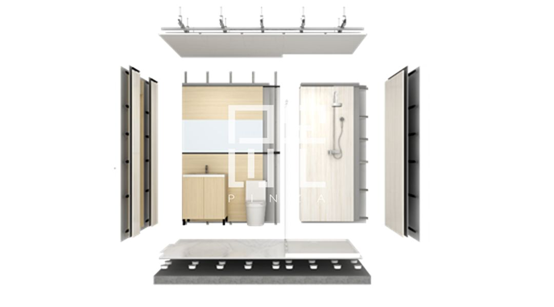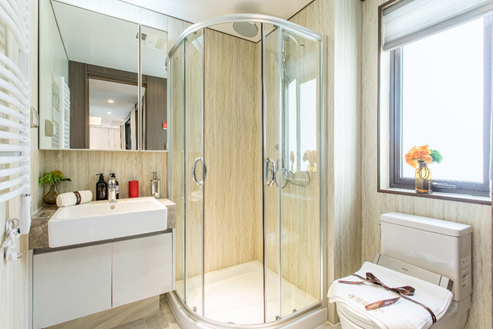△分体装配式卫生间

4、装配化收纳解决方案，全空间，更包容。

收纳是一个居住空间的大课题，大空间通过收纳保持整洁优雅，小空间通过收纳让活动空间最大化。本案用装配式科技内装体系，为各个细分空间量身定制解决方案，在环保、承重、耐脏、易洁、抗菌等方面都坚持高标准，为一家人的温馨生活提供安全、省心、健康、舒适。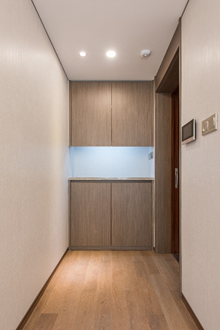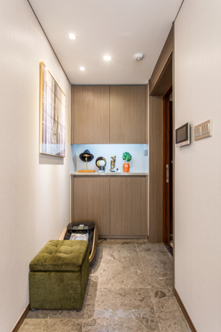△玄关、阳台

5、于细微之处见真章

品宅装配式科技内装可以实现整屋部装产品的工业化生产，去手工化，最大程度杜绝误差，同时，品宅也通过专利技术把细节处理到尽善尽美。

品宅的装配式灯带檐口体系，在与墙面交接的阴角和泛光灯槽檐口均采用专利成品配件，平直美观、永不开裂。门、窗套线采用防水的木塑和铝合金材质，质地兼顾，美观华丽，经久耐用。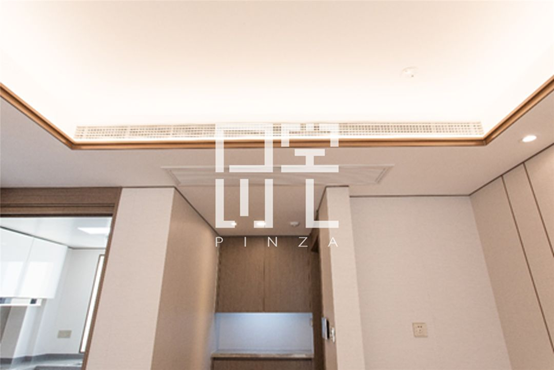△客厅吊顶泛光灯槽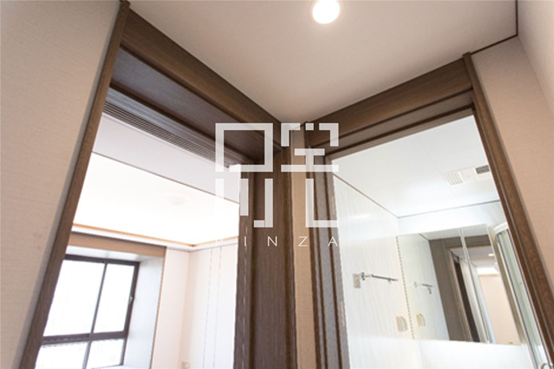△室内门套

政策红利

装配式科技内装在项目上的应用符合政策导向，可直接获取政府补贴/奖励。

产品溢价

使用装配化工艺体系的产品达到内装无醛环保最高标准，以极致健康、安全、环保的居住体验，拉大与当前精装修市场的差距，竞争优势明显，产品溢价能力强。

工期极短

采用自有的BIM设计平台，丰富部品、庞大族库、多样组合、灵活调控、秒速出图，全流程信息化管控，高效实现工业化生产、流水线装配，工期缩短为传统做法的50%，普通住宅10天就能装好一套。

成本减低

人工成本降低50%

时间成本降低50%

维修成本降低80%

品质保证

装配式科技内装体系以内装工业化革新装修方式，去手工化、去复杂化，在标准化生产的基础上更可实现定制化解决方案，应用于精装修商品房，可兼顾实用性、灵活性、安全性、美观性、舒适性、可持续性。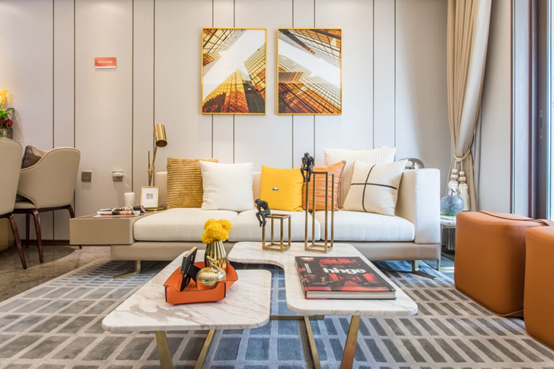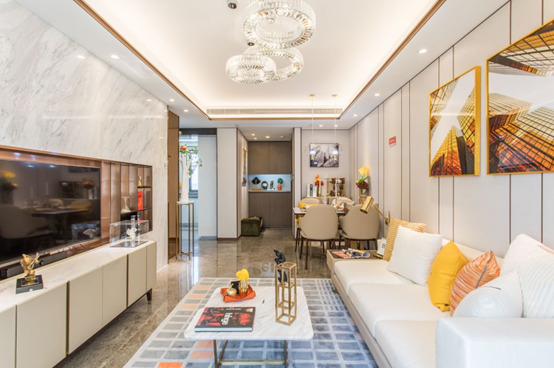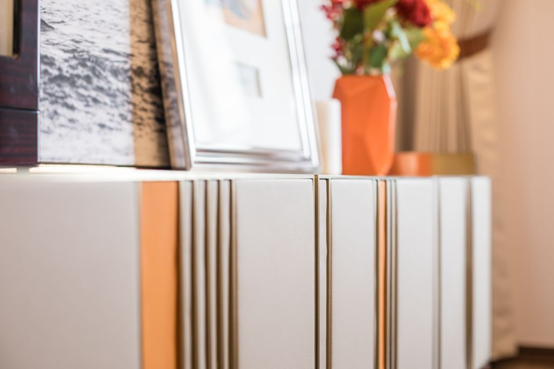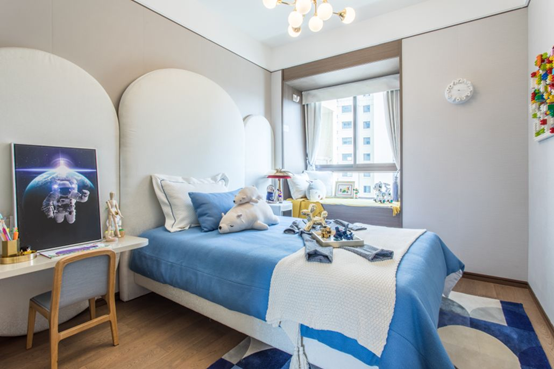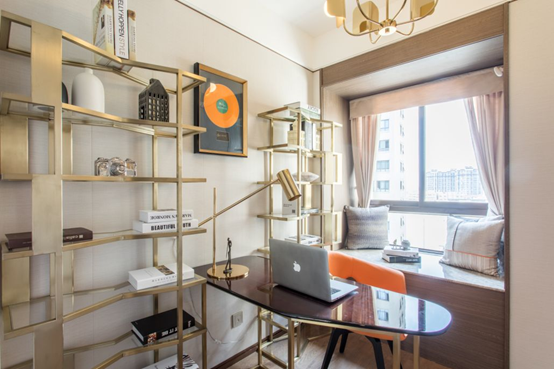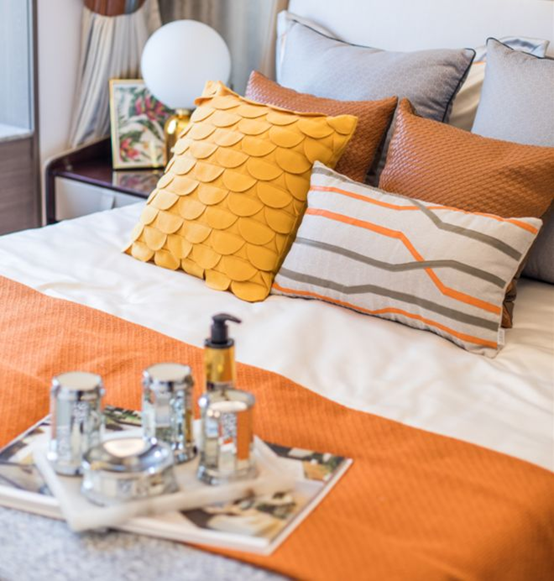`声明：本文由入驻焦点开放平台的作者撰写，除焦点官方账号外，观点仅代表作者本人，不代表焦点立场错误信息举报电话： 400-099-0099，邮箱：jubao@vip.sohu.com，或点此进行意见反馈，或点此进行举报投诉。`A B C D E F G H J K L M N P Q R S T W X Y Z
A - B - C - D - E
• A
• 鞍山
• 安庆
• 安阳
• 安顺
• 安康
• 澳门
• B
• 北京
• 保定
• 包头
• 巴彦淖尔
• 本溪
• 蚌埠
• 亳州
• 滨州
• 北海
• 百色
• 巴中
• 毕节
• 保山
• 宝鸡
• 白银
• 巴州
• C
• 承德
• 沧州
• 长治
• 赤峰
• 朝阳
• 长春
• 常州
• 滁州
• 池州
• 长沙
• 常德
• 郴州
• 潮州
• 崇左
• 重庆
• 成都
• 楚雄
• 昌都
• 慈溪
• 常熟
• D
• 大同
• 大连
• 丹东
• 大庆
• 东营
• 德州
• 东莞
• 德阳
• 达州
• 大理
• 德宏
• 定西
• 儋州
• 东平
• E
• 鄂尔多斯
• 鄂州
• 恩施
F - G - H - I - J
• F
• 抚顺
• 阜新
• 阜阳
• 福州
• 抚州
• 佛山
• 防城港
• G
• 赣州
• 广州
• 桂林
• 贵港
• 广元
• 广安
• 贵阳
• 固原
• H
• 邯郸
• 衡水
• 呼和浩特
• 呼伦贝尔
• 葫芦岛
• 哈尔滨
• 黑河
• 淮安
• 杭州
• 湖州
• 合肥
• 淮南
• 淮北
• 黄山
• 菏泽
• 鹤壁
• 黄石
• 黄冈
• 衡阳
• 怀化
• 惠州
• 河源
• 贺州
• 河池
• 海口
• 红河
• 汉中
• 海东
• 怀来
• I
• J
• 晋中
• 锦州
• 吉林
• 鸡西
• 佳木斯
• 嘉兴
• 金华
• 景德镇
• 九江
• 吉安
• 济南
• 济宁
• 焦作
• 荆门
• 荆州
• 江门
• 揭阳
• 金昌
• 酒泉
• 嘉峪关
K - L - M - N - P
• K
• 开封
• 昆明
• 昆山
• L
• 廊坊
• 临汾
• 辽阳
• 连云港
• 丽水
• 六安
• 龙岩
• 莱芜
• 临沂
• 聊城
• 洛阳
• 漯河
• 娄底
• 柳州
• 来宾
• 泸州
• 乐山
• 六盘水
• 丽江
• 临沧
• 拉萨
• 林芝
• 兰州
• 陇南
• M
• 牡丹江
• 马鞍山
• 茂名
• 梅州
• 绵阳
• 眉山
• N
• 南京
• 南通
• 宁波
• 南平
• 宁德
• 南昌
• 南阳
• 南宁
• 内江
• 南充
• P
• 盘锦
• 莆田
• 平顶山
• 濮阳
• 攀枝花
• 普洱
• 平凉
Q - R - S - T - W
• Q
• 秦皇岛
• 齐齐哈尔
• 衢州
• 泉州
• 青岛
• 清远
• 钦州
• 黔南
• 曲靖
• 庆阳
• R
• 日照
• 日喀则
• S
• 石家庄
• 沈阳
• 双鸭山
• 绥化
• 上海
• 苏州
• 宿迁
• 绍兴
• 宿州
• 三明
• 上饶
• 三门峡
• 商丘
• 十堰
• 随州
• 邵阳
• 韶关
• 深圳
• 汕头
• 汕尾
• 三亚
• 三沙
• 遂宁
• 山南
• 商洛
• 石嘴山
• T
• 天津
• 唐山
• 太原
• 通辽
• 铁岭
• 泰州
• 台州
• 铜陵
• 泰安
• 铜仁
• 铜川
• 天水
• 天门
• W
• 乌海
• 乌兰察布
• 无锡
• 温州
• 芜湖
• 潍坊
• 威海
• 武汉
• 梧州
• 渭南
• 武威
• 吴忠
• 乌鲁木齐
X - Y - Z
• X
• 邢台
• 徐州
• 宣城
• 厦门
• 新乡
• 许昌
• 信阳
• 襄阳
• 孝感
• 咸宁
• 湘潭
• 湘西
• 西双版纳
• 西安
• 咸阳
• 西宁
• 仙桃
• 西昌
• Y
• 运城
• 营口
• 盐城
• 扬州
• 鹰潭
• 宜春
• 烟台
• 宜昌
• 岳阳
• 益阳
• 永州
• 阳江
• 云浮
• 玉林
• 宜宾
• 雅安
• 玉溪
• 延安
• 榆林
• 银川
• Z
• 张家口
• 镇江
• 舟山
• 漳州
• 淄博
• 枣庄
• 郑州
• 周口
• 驻马店
• 株洲
• 张家界
• 珠海
• 湛江
• 肇庆
• 中山
• 自贡
• 资阳
• 遵义
• 昭通
• 张掖
• 中卫

1室1厅1厨1卫1阳台

1
2
3
4
5

0
1
2

1

1

0
1
2
3报名成功，资料已提交审核A B C D E F G H J K L M N P Q R S T W X Y Z
A - B - C - D - E
• A
• 鞍山
• 安庆
• 安阳
• 安顺
• 安康
• 澳门
• B
• 北京
• 保定
• 包头
• 巴彦淖尔
• 本溪
• 蚌埠
• 亳州
• 滨州
• 北海
• 百色
• 巴中
• 毕节
• 保山
• 宝鸡
• 白银
• 巴州
• C
• 承德
• 沧州
• 长治
• 赤峰
• 朝阳
• 长春
• 常州
• 滁州
• 池州
• 长沙
• 常德
• 郴州
• 潮州
• 崇左
• 重庆
• 成都
• 楚雄
• 昌都
• 慈溪
• 常熟
• D
• 大同
• 大连
• 丹东
• 大庆
• 东营
• 德州
• 东莞
• 德阳
• 达州
• 大理
• 德宏
• 定西
• 儋州
• 东平
• E
• 鄂尔多斯
• 鄂州
• 恩施
F - G - H - I - J
• F
• 抚顺
• 阜新
• 阜阳
• 福州
• 抚州
• 佛山
• 防城港
• G
• 赣州
• 广州
• 桂林
• 贵港
• 广元
• 广安
• 贵阳
• 固原
• H
• 邯郸
• 衡水
• 呼和浩特
• 呼伦贝尔
• 葫芦岛
• 哈尔滨
• 黑河
• 淮安
• 杭州
• 湖州
• 合肥
• 淮南
• 淮北
• 黄山
• 菏泽
• 鹤壁
• 黄石
• 黄冈
• 衡阳
• 怀化
• 惠州
• 河源
• 贺州
• 河池
• 海口
• 红河
• 汉中
• 海东
• 怀来
• I
• J
• 晋中
• 锦州
• 吉林
• 鸡西
• 佳木斯
• 嘉兴
• 金华
• 景德镇
• 九江
• 吉安
• 济南
• 济宁
• 焦作
• 荆门
• 荆州
• 江门
• 揭阳
• 金昌
• 酒泉
• 嘉峪关
K - L - M - N - P
• K
• 开封
• 昆明
• 昆山
• L
• 廊坊
• 临汾
• 辽阳
• 连云港
• 丽水
• 六安
• 龙岩
• 莱芜
• 临沂
• 聊城
• 洛阳
• 漯河
• 娄底
• 柳州
• 来宾
• 泸州
• 乐山
• 六盘水
• 丽江
• 临沧
• 拉萨
• 林芝
• 兰州
• 陇南
• M
• 牡丹江
• 马鞍山
• 茂名
• 梅州
• 绵阳
• 眉山
• N
• 南京
• 南通
• 宁波
• 南平
• 宁德
• 南昌
• 南阳
• 南宁
• 内江
• 南充
• P
• 盘锦
• 莆田
• 平顶山
• 濮阳
• 攀枝花
• 普洱
• 平凉
Q - R - S - T - W
• Q
• 秦皇岛
• 齐齐哈尔
• 衢州
• 泉州
• 青岛
• 清远
• 钦州
• 黔南
• 曲靖
• 庆阳
• R
• 日照
• 日喀则
• S
• 石家庄
• 沈阳
• 双鸭山
• 绥化
• 上海
• 苏州
• 宿迁
• 绍兴
• 宿州
• 三明
• 上饶
• 三门峡
• 商丘
• 十堰
• 随州
• 邵阳
• 韶关
• 深圳
• 汕头
• 汕尾
• 三亚
• 三沙
• 遂宁
• 山南
• 商洛
• 石嘴山
• T
• 天津
• 唐山
• 太原
• 通辽
• 铁岭
• 泰州
• 台州
• 铜陵
• 泰安
• 铜仁
• 铜川
• 天水
• 天门
• W
• 乌海
• 乌兰察布
• 无锡
• 温州
• 芜湖
• 潍坊
• 威海
• 武汉
• 梧州
• 渭南
• 武威
• 吴忠
• 乌鲁木齐
X - Y - Z
• X
• 邢台
• 徐州
• 宣城
• 厦门
• 新乡
• 许昌
• 信阳
• 襄阳
• 孝感
• 咸宁
• 湘潭
• 湘西
• 西双版纳
• 西安
• 咸阳
• 西宁
• 仙桃
• 西昌
• Y
• 运城
• 营口
• 盐城
• 扬州
• 鹰潭
• 宜春
• 烟台
• 宜昌
• 岳阳
• 益阳
• 永州
• 阳江
• 云浮
• 玉林
• 宜宾
• 雅安
• 玉溪
• 延安
• 榆林
• 银川
• Z
• 张家口
• 镇江
• 舟山
• 漳州
• 淄博
• 枣庄
• 郑州
• 周口
• 驻马店
• 株洲
• 张家界
• 珠海
• 湛江
• 肇庆
• 中山
• 自贡
• 资阳
• 遵义
• 昭通
• 张掖
• 中卫• 手机• 分享
• 设计
免费设计
• 计算器
装修计算器
• 入驻
合作入驻
• 联系
联系我们
• 置顶
返回顶部